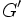# Equivalence of definitions of LUCS-Baer Lie group

This article gives a proof/explanation of the equivalence of multiple definitions for the term LUCS-Baer Lie group
View a complete list of pages giving proofs of equivalence of definitions

## Statement

Suppose$G$ is a group of nilpotency class two (i.e., its derived subgroup is contained in ts center). Then, the following are equivalent:

1. Every element of the derived subgroup$G'$ has a unique square root in$G$.
2. Every element of its derived subgroup$G'$ has a unique square root among the elements in the center$Z(G)$.
3. Every element of the derived subgroup$G'$ has a unique square root in$G$ and that square root is in the center$Z(G)$.

## Proof

### (3) implies (1)

This is immediate.

### (3) implies (2)

If the square root is unique in all of$G$ and also happens to be in the center, it must be unique among the elements of the center as well.

### (1) implies (3)

Given: A group$G$, an element$g \in G'$ such that there is a unique element$x \in G$ satisfying$x^2 = g$.

To prove:$x \in Z(G)$

Proof: Since$G$ has nilpotency class at most two,$g \in G' \le Z(G)$, so$g \in Z(G)$. The uniqueness of the square root, combined with Fact (1), tells us that$x \in Z(G)$ as well.

### (2) implies (3)

Given: A group$G$ with the property that every element of$G'$ has a unique square root in$Z(G)$. An element$g \in G'$ with square root$x \in Z(G)$.

To prove:$x$ is the unique square root of$g$ in all of$G$.

Proof: Since every element of$G'$ has a unique square root in$Z(G)$, this in particular implies that the identity element has a unique square root in$Z(G)$, so$Z(G)$ is 2-torsion-free. By Fact (2) combined with$G$ being nilpotent, this implies that the squaring map is injective from$G$ to itself, so$x$ is the unique square root of$g$ in all of$G$.